null 机器学习之线性回归 - K码农

# 1|0模型表示

$\begin{array}{}\text{(1)}& y=b+\sum _{i=1}^{n}{\theta }_{i}{x}_{i}\end{array}$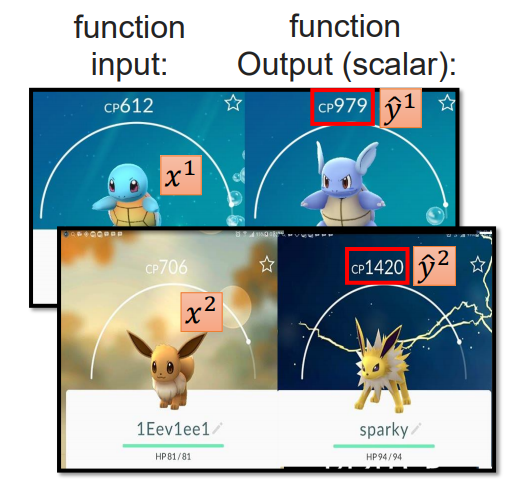$\begin{array}{}\text{(2)}& y=\sum _{i=0}^{n}{\theta }_{i}{x}_{i}\end{array}$

# 2|0模型评估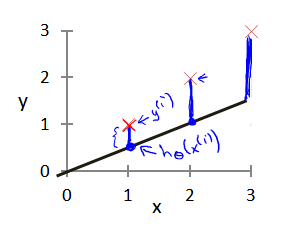$\begin{array}{}\text{(3)}& L\left(\theta \right)=\frac{1}{2m}\sum _{i=1}^{m}\left({h}_{\theta }\left({x}^{\left(i\right)}\right)-{y}^{\left(i\right)}{\right)}^{2}\end{array}$

def compute_cost(X, y, theta):
inner = np.power(((X * theta.T) - y), 2)
return np.sum(inner) / (2 * len(X))

# 3|0模型学习

$\begin{array}{}\text{(4)}& {\theta }^{\ast }& =\mathrm{arg}\phantom{\rule{thickmathspace}{0ex}}\underset{\theta }{min}L\left(\theta \right)\text{(5)}& & =\mathrm{arg}\phantom{\rule{thickmathspace}{0ex}}\underset{\theta }{min}\frac{1}{2m}\sum _{i=1}^{m}\left({h}_{\theta }\left({x}^{\left(i\right)}\right)-{y}^{\left(i\right)}{\right)}^{2}\end{array}$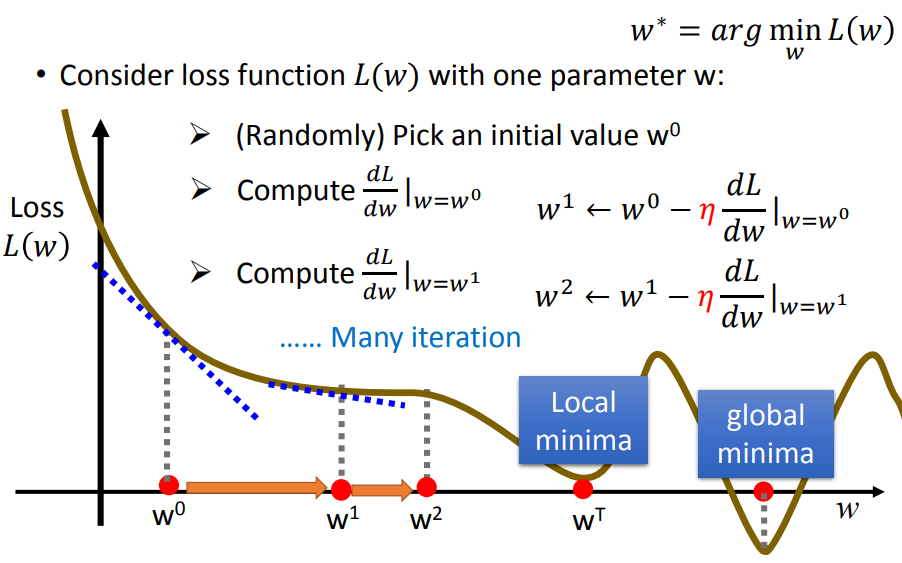$\begin{array}{}\text{(6)}& \frac{\mathrm{\partial }L}{\mathrm{\partial }{\theta }_{j}}& =\frac{1}{2m}\sum _{i=1}^{m}2\cdot \left({h}_{\theta }{x}^{\left(i\right)}-{y}^{\left(i\right)}\right)\cdot {x}_{j}^{\left(i\right)}\text{(7)}& & =\sum _{i=1}^{m}\left({h}_{\theta }{x}^{\left(i\right)}-{y}^{\left(i\right)}\right)\cdot {x}_{j}^{\left(i\right)}\end{array}$

$\begin{array}{}\text{(8)}& {\theta }_{j}^{\left(1\right)}& ={\theta }_{j}^{\left(0\right)}-\alpha \cdot \frac{\mathrm{\partial }L}{\mathrm{\partial }{\theta }_{j}}\text{(9)}& & ={\theta }_{j}^{\left(0\right)}-\alpha \cdot \sum _{i=1}^{m}\left({h}_{\theta }{x}^{\left(i\right)}-{y}^{\left(i\right)}\right)\cdot {x}_{j}^{\left(i\right)}\end{array}$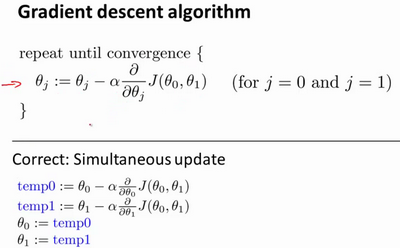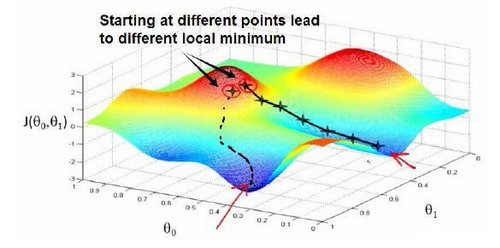# 4|0模型总结

## 4|1算法流程

1. 根据特征维度随机初始化一组参数 $\left({\theta }_{0},{\theta }_{1},\cdots ,{\theta }_{n}\right)$$(\theta_0,\theta_1,\cdots,\theta_n)$
2. 根据该参数计算所有样本的预测值 ${h}_{\theta }\left(x\right)$$h_{\theta}(x)$
3. 根据公式(9)更新权重参数；
4. 重复执行步骤2和步骤3，直到权重参数收敛或者达到一定的迭代次数。

## 4|2存在的问题

• 只适用于解决线性可分的问题，因为目标函数是多种变量的线性组合；
• 对异常值敏感

__EOF__